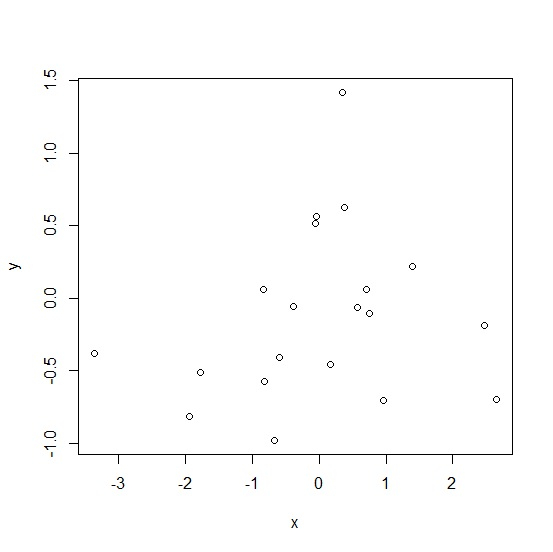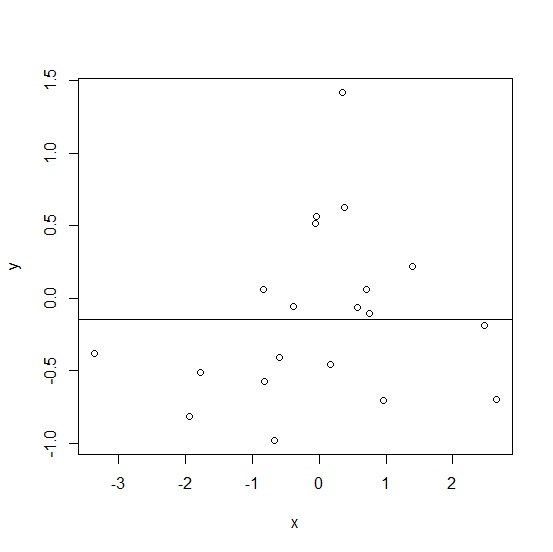# How to create horizontal line for Y variable at median in base R plot?

To create horizontal line for Y variable at median in base R plot, we can follow the below steps −

• First of all, create two vectors and plot them.
• Create the horizontal line at median using abline function.

## Create the vectors and plot them

Let’s create two random vectors and plot them as shown below −

### Example

Live Demo

x<-rnorm(20)
y<-rnorm(20)
plot(x,y)

On executing, the above script generates the below output(this output will vary on your system due to randomization) −

### Output## Create the horizontal line at median

Use abline function with h argument to create the horizontal line at median as shown below −

### Example

Live Demo

x<-rnorm(20)
y<-rnorm(20)
plot(x,y)
abline(h=median(y))

### Output